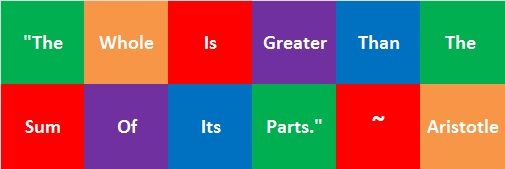# 433 The Whole Is Greater Than the Sum of Its Parts

Today I practiced a song with a choir comprised of nine smaller choirs. Every time before I had only practiced the song with our smaller choir. As I listened to all the voices in this big choir while we sang in harmony, I thought, “A big choir sounds SO good. The whole is greater than the sum of its parts!”Here is a professional recording of the beautiful song we are singing. The music in this youtube video is directed by the composer, Rob Gardner. It features the London Symphony Orchestra at Air Studios, London and the Spire Choir at Camelback Baptist Church in Paradise Valley, Arizona.

The number 433 is also greater than the sum of its parts.

12² + 17² = 433

A Chinese checker board looks like a star and has 121 holes so 121 is called a star number. The first star number is 1, followed by 13, 37, 73, and 121. The ninth star number is 433.

433 is one more than twelve times the eighth triangular number. Star numbers and triangular numbers are related like that.

145-408-433 is a primitive Pythagorean triple.

Here is the factoring information for the number 433:

• 433 is a prime number. 431 and 433 are twin primes.
• Prime factorization: 433 is prime.
• The exponent of prime number 433 is 1. Adding 1 to that exponent we get (1 + 1) = 2. Therefore 433 has exactly 2 factors.
• Factors of 433: 1, 433
• Factor pairs: 433 = 1 x 433
• 433 has no square factors that allow its square root to be simplified. √433 ≈ 20.8087How do we know that 433 is a prime number? If 433 were not a prime number, then it would be divisible by at least one prime number less than or equal to √433 ≈ 20.8087. Since 433 cannot be divided evenly by 2, 3, 5, 7, 11, 13, 17, or 19, we know that 433 is a prime number.